##### PostgreSQL regexp_split_to_array() Function

In Postgresql, the regexp_split_to_array() function is used to split the specified string into an array using the specified POSIX regular expressions. The strings as well as the POSIX regular expression are provided to the function as an argument. This expression will act as a separator for the string elements in the array. A flag can also be specified in the function. This function works the same as the string_to_array() function.

Let’s get deep into the details of the regexp_split_to_array() function.

## PostgreSQL regexp_split_to_array() Function

As discussed earlier, the the regexp_split_to_array() function splits the given string into an array placing the regular expressions as separators. There also exists a third argument, which is completely optional, that can control some specific behavior of the function. The function returns an array. Let's have a look at the basic syntax of the function:

`regexp_split_to_array(String,   Regexp[, Flag]);`

In the above syntax:

● The String is the main string that needs to be split.

● The second argument, Regexp, is the POSIX reg expression that will act as a separator.

● The third argument is the flag that can slightly mold the functionality of the function. For example ”i” flag will consider the sensitive matching.

The function returns an array that contains the string split through the regular expressions as separators. Below are some of the important things about the value of Regex:

● If the Regex is NULL the function will also return NULL.

● If the value of Regex is an empty string, the function will simply return all the characters of the original string.

Let’s move on to the examples of the regexp_split_to_array() function to understand it better.

Example 1

Consider the following query as an example:

`SELECT regexp_split_to_array('bats   Eaten gate Atone', '\s+');`

In this query, the string, 'bats Eaten gate Atone', will be separated by whitespaces as the regular expression is ”\s+” which gives the white spacing between the string elements. The output of the query is: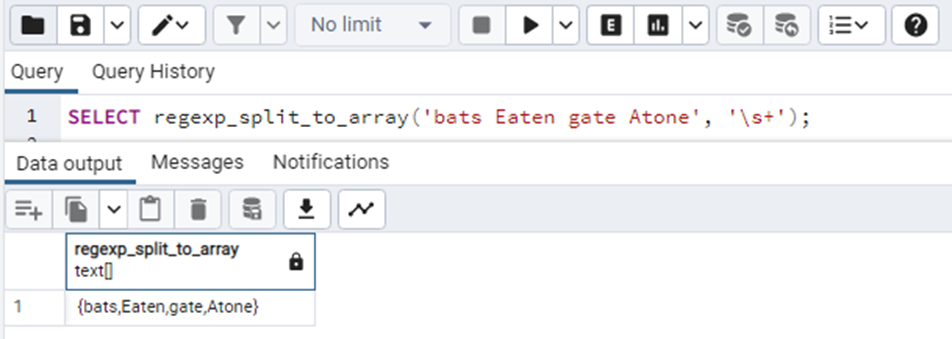Consider the case where the Regex is an empty string. The query is as follows:

`SELECT regexp_split_to_array('bats   Eaten gate Atone', '');`

The output of this query will be separating all the characters of the string like this: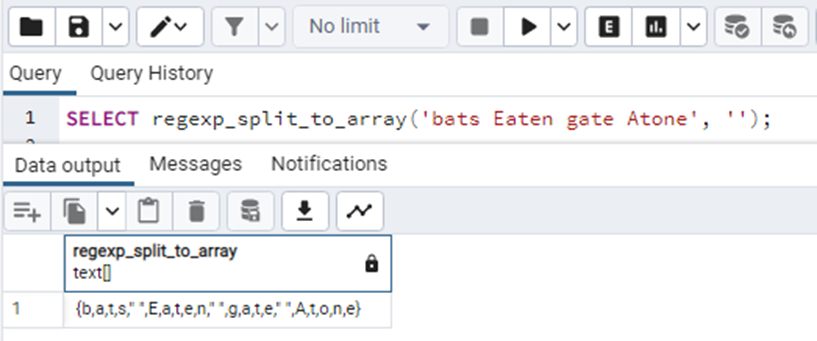Now consider using the optional flags in the query. In the below query, we will be using the “i” flag.

`SELECT regexp_split_to_array('bats Eaten gate Atone', 'at.', 'i');`

The “i” flag will match the regex for the case-insensitive characters in the string and then accordingly return the value like this: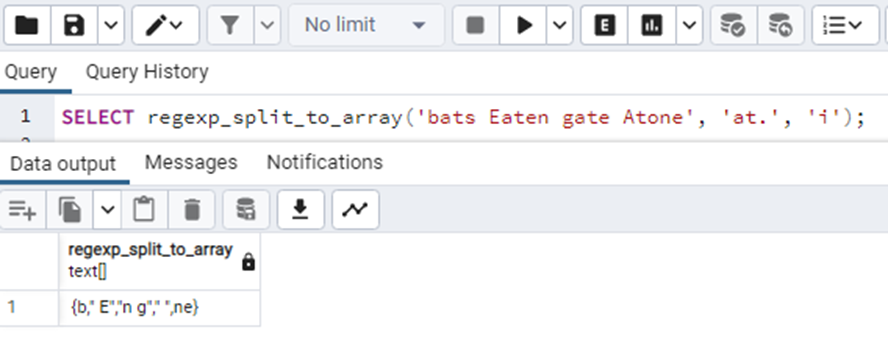In the above output, the” i” flag has found “at” in the string and its next character(represented by “.”) and separated them by commas. The double quotes “ ” are for spaces.

Example 2

We can also use this function with the tables. Let's see how it works:

Consider the following table project_status having 4 columns: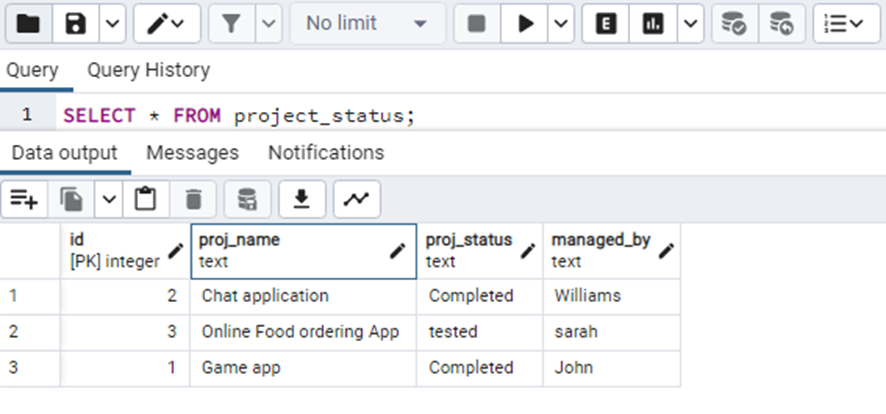Now if we want to apply the function regexp_split_to_array() on the proj_name column, we will have to write the following query:

```SELECT regexp_split_to_array(proj_name, '\s+')
FROM   project_status;```

This query will separate the proj_name strings into the array elements having the data type TEXT. the output will be: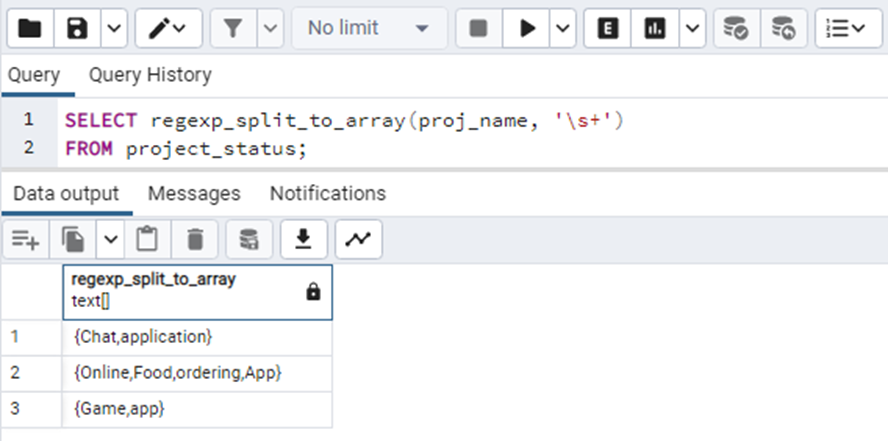The above output clearly proves that the function has been executed successfully and works the same in the case of tables too.

This was all about the regexp_split_to_array() function.

Conclusion

This function works the same as the string_to_array() function does. The function takes the string, the regexp, and the flag(if any) as arguments and returns an array that contains the string elements/characters with the Regexp as separators. In this article, we have learned about the regexp_split_to_array() function in PostgreSQL.